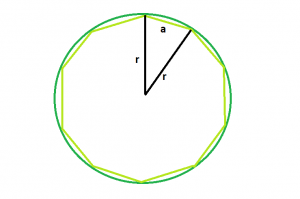# Program to find the Circumcircle of any regular polygon

Given a n-sided polygon with side length a. The task is to find the area of the cicumcircle of the polygon.

Examples:

```Input: n = 10, a = 3
Output: 1.99737

Input: n = 5, a = 6
Output: 3.02487
```

## Recommended: Please try your approach on {IDE} first, before moving on to the solution.Approach: A regular n-gon divides the circle into n pieces, so the central angle of the triangle is a full circle divided by n: 360 deg/n.
Applying the law of cosines for the three side lengths of the triangle, we get

c2 = a2 + b2 – 2ab cos C
or, a2 = r2 + r2 – 2rr cos (360/n)
or, a2 = 2r2 – 2r2 cos (360/n)
or, c2 = r2 (2 – 2 cos (360/n))
so, a=r√(2-2cos(360/n))

Therefore,
r=a/√(2-2cos(360/n))

Below is the implementation of the above approach:

## C++

 `// C++ Program to find the radius ` `// of the circumcircle of the given polygon ` ` `  `#include ` `using` `namespace` `std; ` ` `  `// Function to find the radius ` `// of the circumcircle ` `float` `findRadiusOfcircumcircle(``float` `n, ``float` `a) ` `{ ` ` `  `    ``// these cannot be negative ` `    ``if` `(n < 0 || a < 0) ` `        ``return` `-1; ` ` `  `    ``// Radius of the circumcircle ` `    ``float` `radius = a / ``sqrt``(2 - (2 * ``cos``(360 / n))); ` ` `  `    ``// Return the radius ` `    ``return` `radius; ` `} ` ` `  `// Driver code ` `int` `main() ` `{ ` ` `  `    ``float` `n = 5, a = 6; ` ` `  `    ``// Find the radius of the circumcircle ` `    ``cout << findRadiusOfcircumcircle(n, a) << endl; ` ` `  `    ``return` `0; ` `} `

## Java

 `// Java Program to find the radius ` `// of the circumcircle of the given polygon ` ` `  `import` `java.io.*; ` ` `  `class` `GFG { ` `   `  `// Function to find the radius ` `// of the circumcircle ` `static` `float` `findRadiusOfcircumcircle(``float` `n, ``float` `a) ` `{ ` ` `  `    ``// these cannot be negative ` `    ``if` `(n < ``0` `|| a < ``0``) ` `        ``return` `-``1``; ` ` `  `    ``// Radius of the circumcircle ` `    ``float` `radius = (``float``)(a / Math.sqrt(``2` `- (``2` `* Math.cos(``360` `/ n)))); ` ` `  `    ``// Return the radius ` `    ``return` `radius; ` `} ` ` `  `// Driver code ` ` `  `    ``public` `static` `void` `main (String[] args) { ` `        ``float` `n = ``5``, a = ``6``; ` ` `  `    ``// Find the radius of the circumcircle ` `    ``System.out.println( findRadiusOfcircumcircle(n, a)) ; ` ` `  `    ``} ` `} ` `// This code is contributed  ` `// by anuj_67.. `

## Python 3

 `# Python3 Program to find the  ` `# radius of the circumcircle  ` `# of the given polygon  ` ` `  `# from math import all methods ` `from` `math ``import` `*` ` `  `# Function to find the radius  ` `# of the circumcircle  ` `def` `findRadiusOfcircumcircle(n, a) : ` ` `  `    ``# these cannot be negative ` `    ``if` `n < ``0` `or` `a < ``0` `: ` `        ``return` `-``1` ` `  `    ``# Radius of the circumcircle ` `    ``radius ``=` `a ``/` `sqrt(``2` `-` `(``2` `*` `cos(``360` `/` `n))) ` ` `  `    ``# Return the radius ` `    ``return` `radius ` ` `  `# Driver code ` `if` `__name__ ``=``=` `"__main__"` `: ` ` `  `    ``n , a ``=` `5``, ``6` ` `  `    ``# Find the radius of the circumcircle  ` `    ``print``(``round``(findRadiusOfcircumcircle(n, a), ``5``)) ` ` `  `# This code is contributed  ` `# by ANKITRAI1 `

## C#

 `// C# Program to find the radius ` `// of the circumcircle of the given polygon ` `using` `System; ` ` `  `class` `GFG  ` `{ ` ` `  `// Function to find the radius ` `// of the circumcircle ` `static` `float` `findRadiusOfcircumcircle(``float` `n,  ` `                                      ``float` `a) ` `{ ` ` `  `    ``// these cannot be negative ` `    ``if` `(n < 0 || a < 0) ` `        ``return` `-1; ` ` `  `    ``// Radius of the circumcircle ` `    ``float` `radius = (``float``)(a / Math.Sqrt(2 -  ` `                   ``(2 * Math.Cos(360 / n)))); ` ` `  `    ``// Return the radius ` `    ``return` `radius; ` `} ` ` `  `// Driver code ` `public` `static` `void` `Main ()  ` `{ ` `    ``float` `n = 5, a = 6; ` ` `  `    ``// Find the radius of the circumcircle ` `    ``Console.WriteLine(findRadiusOfcircumcircle(n, a)); ` `} ` `} ` ` `  `// This code is contributed  ` `// by anuj_67 `

## PHP

 ` `

Output:

```3.02487
```

Attention reader! Don’t stop learning now. Get hold of all the important DSA concepts with the DSA Self Paced Course at a student-friendly price and become industry ready.

My Personal Notes arrow_drop_upCheck out this Author's contributed articles.

If you like GeeksforGeeks and would like to contribute, you can also write an article using contribute.geeksforgeeks.org or mail your article to contribute@geeksforgeeks.org. See your article appearing on the GeeksforGeeks main page and help other Geeks.

Please Improve this article if you find anything incorrect by clicking on the "Improve Article" button below.

Improved By : vt_m, AnkitRai01

Article Tags :
Practice Tags :

Be the First to upvote.

Please write to us at contribute@geeksforgeeks.org to report any issue with the above content.